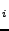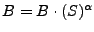Next: UNWRAP Up: Implementation Previous: spectral   Contents

## goldstein

The algorithm is implemented as:

• Read in buffer Bof PF_BLOCKSIZE lines (overlap).
• Get block B=Bas input block, see Fig. 29.9.
• B=fft2d(B) (obtain complex spectrum)
• A=abs(B) (magnitude of spectrum)
• S=smooth(A) (convolution with kernel)
• S=S/max(S) (S between 0 and 1)
•(weight complex spectrum)
• B=ifft2d(B) (result in space domain)
• If all blocks of buffer done, write to disk.For a block [pixlo:pixhi], e.g., [0:15], the output equals for an overlap (=3), [pixlo+overlap:pixhi-overlap], [3:12]. The number of output equals size-2overlap = pixhi-pixlo+1-2overlap = 10.

Leijen 2009-04-14